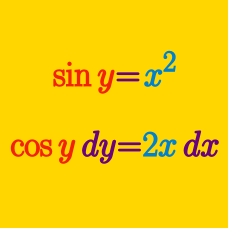Calculus

Implicit Differentiation - Polynomials

What is the slope of the curve $8x^2 + 5y^2 = r^2$ (where $r \neq 0)$ at a point $(x_{1}, y_{1})$ that lies on the curve?

What is the slope of the tangent line of the curve $x^2 = y^2$ at the point $(15, -15)?$

If $y^{4}=17x,$ what is $\displaystyle \frac{dy}{dx}?$

Determine the slope of the line tangent to the circle $(x-2)^2 + (y-4)^2 = 32$ at the point $(x,y) =(6,0)$.

If $y^3-4y^2=x^5+3x^4,$ what is $\displaystyle \frac{dy}{dx}?$

×